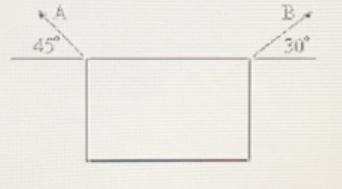# Problem: A 10-kg sign is held by two ropes as shown in the figure below. What is the tension on rope A? a. 44 N b. 88 N c. 69 N d. 98 N e. 72 N

###### FREE Expert Solution

Weight, W = mg

The weight of the sign is given by:

W= mg = (10)(9.8) = 98 N.

In the horizontal direction, the forces are:

- TAcos(45°) + TB cos (30°) = 0

Along the vertical direction:

TA sin (45°) + TB sin (30°) - Ws = 0

81% (107 ratings)###### Problem Details

A 10-kg sign is held by two ropes as shown in the figure below. What is the tension on rope A?a. 44 N

b. 88 N

c. 69 N

d. 98 N

e. 72 N